# Voltage and current | What are they?

Voltage and current are two very important quantities that we want to monitor in circuits to exactly know what’s going on.What you see is a voltmeter on the left, used to measure voltage, and an ammeter on the right, which measures current.

But what are they, and what is the difference between the two and their relationship?

### Voltage

Voltage (symbol V) is measured between two points, and it is the work done to move electric charge (electrons) from the more negative point (i.e. lower potential) to the more positive point (i.e. higher potential), and because of this definition it is also called potential difference or electromotive force (EMF).

Its unit of measure is the volt (V).

#### Electron flowElectrons have negative electric charge, hence they move towards the positive point (higher potential).

Voltages are generated by doing work on charges in devices such as: batteries, in which electrochemical energy is converted; solar cells (photovoltaic conversion of the energy of photons); generators, that convert mechanical energy by magnetic force, etc.

Mathematically, voltage is defined as the derivative of work W (measured in joules, J) with respect to charge q, (measured in coulombs, C, which is the unit of measure of electric charge).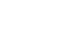#### Overvoltage | EffectsIt’s really important to respect voltage ratings of electronic devices: your phone charges with 5 V, which means if you “give” less, it won’t charge or it will but much more slowly; on the other hand, you will end up destroying it if you supply a higher voltage. Phone, tablet and laptop chargers work with a way higher voltage than 5 V, usually 110 V or 220 V (depending on your country, the outlet voltage will be different), but it’s completely fine for your devices because chargers have a circuit that lowers tension to the desired voltage.

### Current

Current (symbol I) is the rate of flow of electric charge past a point and it’s measured in amperes or amps (symbol A); therefore, a current of 1 A equals a flow of 1 C of charge per second, that is the derivative of charge q with respect to time t: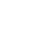#### Conventional current flowBy convention, current in a circuit is considered to flow from a more positive point to a more negative point, even though the actual electron flow is in the opposite direction.

#### Kirchhoff’s current law

Charge is always conserved, and since current is the rate of flow of charge, the sum of the currents into a point (referred to as “node“) in a circuit equals the sum of the currents out, and this is called Kirchhoff’s current law (KCL).I3 is equal to the sum of the two currents I1 and I2 flowing into the node.

### Voltage and current | Differences and relationship

It should now be clear what the main difference between voltage and current is: currents flow through things, whereas voltages are applied across things. Also, we have current flowing through a conductor when we apply a voltage to it, but this is because there is a third physical dimention we need to take into account, and that is resistance.

#### Electric resistance and Ohm’s Laws

To understand the concept of electric resistance, let’s consider a hose: when water flows through it, there is a certain pressure at which the fluid comes out: that is the equivalent of the voltage applied to a wire; the flow of water is analogous to that of electrons, and that’s the current.

If the hose is kinked/narrowed at a point, water will flow more slowly, depending on how much the section gets reduced; this is exactly what happens to current through a wire made out of a conductive material like copper: this decrease in electron flow is to be attributed to a property of that material known as resistivity, which in turn creates resistance that opposes to the flow of electric current.

##### First Ohm’s law

Electric resistance is given by a very important relationship between voltage and current, expressed by the first Ohm’s law, which states that current is directly proportional to the voltage and inversely proportional to the resistance of the conductor/circuit. The formula is: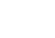from which we also get: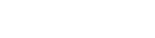The unit of electric resistance is the ohm (symbol: the capital Greek letter omega, Ω).

##### Electric resistivity | Second Ohm’s law

A conductor can be made out of different materials, like copper and aluminium, that have a property called resistivity, measured in Ωm (ohm metre), which measures how strongly it resists electric current.Copper, gold and silver are all metals with very low electric resistivity, meaning their resistance against current is weak; this is why they are widely used in electronics, especially in PCs as they require extremely fast data transfer.

If we want to know the electric resistance of a wire made out of copper (ρ = 1.68×10−8 Ωm at 20 °C), we must also take into account its length l (in metres) and thickness A (in m3), as shown in the formula: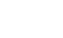As you can see, the thinner the wire, the higher the resistance, and this is the analogy we made with the hose.Depending on the ratings (maximum voltage and current), wires come in numerous thicknesses, each suitable for a specific application: the thicker they are, the more voltage and current they can withstand; for this reason you see mostly thin wires around you, whereas, say, an industrial electric oven will use connections with a much larger diameter.
##### Joule’s effect

When current flows through conductors, these tend to heat up due to Joule’s effect.Incandescent lightbulb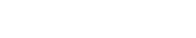#### Voltage and current sources

##### Ideal voltage source

A perfect voltage source is a two-terminal device that mantains a fixed voltage drop across its terminals, regardless of load resistance. A real voltage source can supply only a finite maximum current, and it only behaves like a perfect voltage source with a small resistance connected in series.

##### Ideal current source

A perfect current source is a two-tereminal device that mantains a constant current through the external circuit, regardless of load resistance or applied voltage, but this means it must be capable of supplying any necessary voltage, and real current sources have a limit to the voltage they can provide.

### Power

In physics, power is the work W done over a period of time t, and more specifically the derivative of work with respect to time: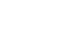and it’s measured in watts (W).

#### Power consumed and power dissipated | Heat

As defined before,

If we multiply voltage by current, we see dq cancels out: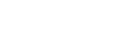This is simply energy/charge × charge/time; we are left with: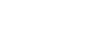and that’s the definition of power; hence,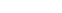Therefore, the power consumed by a circuit/device is equal to the voltage (in volts) multiplied by the current (in amps).

From this, we get: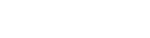So, for example, the current flowing through a 110 W lightbulb running on 220 V is 110 W / 220 V = 0.5 A. Joule’s effect is very noticeable here, since incandescent lightbulbs are designed to convert power into heat which releases light.

From the formula P = VI, you can see that as the current I increases, the power P increases as well and, depending on the circuit, this power can become more or less dissipated. Think about PCs:

Since we talked about resistance, we can see how the amount of power generated in a circuit also depends on this dimension; let’s consider the two formulas:

We can substitute the V in the first equation with RI: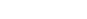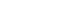As you can see, as R increases, P increases too, while mantaining I constant, and it also gets bigger as I increases while keeping R constant.

Because of this relationship between current and power dissipated, we can talk about overcurrent and overload.

#### Overcurrent and overload | Effects

This is why fuses are used in electronic devices: they are small glass tubes (although there are more types, even in plastic) with a thin filament inside them, which breaks when the rated voltage and current are exceeded.

The most important and mandatory protections against overcurrent and overload, though, are breakers (those kind of switches you have at home that may pop during a thunderstorm or when you are using too many devices all at once): they interrupt voltage and current flow in order to prevent major damages to your house’s electric system.Breakers; you can also switch them off to stop voltage and current when you need to bring modifications to your wirings in order to avoid electric shock.

### Oscilloscopes | AC vs DC and how to “see” them

An extremely useful tool for monitoring circuits and therefore voltage and current is an oscilloscope;

What you see on the right is a DC signal, short for direct current; it is characterised by steady voltage and current, and an example is the output of a battery.

What you see on the left is a sine wave, i.e. a function whose values oscillate according to the formula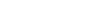This is defined as AC, short for alternating current. AC is therefore a signal in which voltage and current cover all values from –V0 and –I0 to +V0 and +I0.